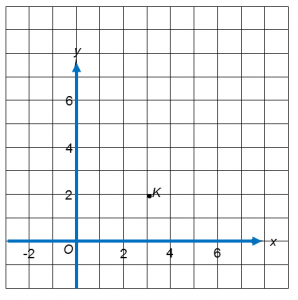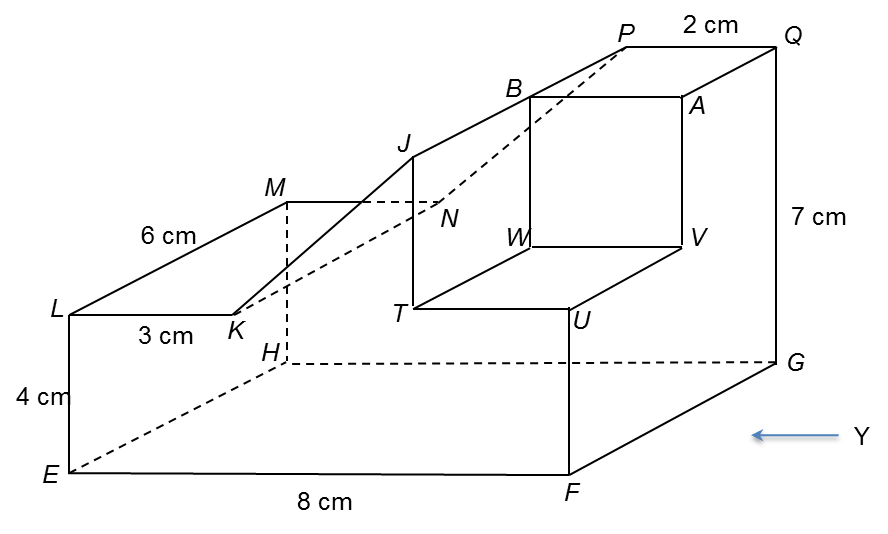# SPM Mathematics (Model Test Paper) – Paper 2 Section B (Question Only)

SPM Mathematics (Model Test Paper)

Section B
[48 marks]
Answer any four questions from this section.
12 (a) Complete Table 1 in the answer space for the equation y = 2x2− 8x + 1.  [2 marks]
(b) For this part of the question, use the graph paper provided. You may use a flexible curve rule. By using a scale of 2 cm to 1 unit of the x-axis and 2 cm to 5 units on the y-axis, draw the graph of y = 2x2− 8x + 1 for 0 ≤ x ≤ 6. [4 marks]
(i) the value of y when x = 3,
(ii) the value of x when y = 2,  [2 marks]
(d)   Draw a suitable straight line on your graph to find all the values of x which satisfy the equation 2x2− 4x − 11 = 0 for 0 ≤ x ≤ 6. State the values of x.   [4 marks]

(a)
 x 0 0.5 1 2 3 4 5 5.5 6 y 1 −2.5 −5 −7 −5 1 25
Table 1

13. (a)  Diagram 8.1 shows point K ( 3, 2 ) marked on a Cartesian plane.Diagram 8.1

The transformation T is a translation ( −2  3 ).
The transformation R is a reflection in the line y = 3.
State the coordinates of the image of point K under each of the following transformations.
(i) T
(ii) TR    [3 marks]
(b)  Diagram 8.2  shows three trapeziums, ABCD, PQRS and PTUV drawn on a Cartesian plane.Diagram 8.2

(i) Trapezium PTUV is the image of trapezium ABCD under the combined transformation VW
Describe in full, the transformation W and the transformation V.  [ 6 marks]
(ii) Given that the area of trapezium ABCD is 14 cm2, calculate the area, in cm2, of the shaded region. [3 marks]

14.  The data in Diagram 9 shows the weight of meat, in kg, sold each day over 40 days.

 36 63 43 47 67 71 68 79 92 49 41 52 66 78 84 55 77 86 58 94 60 74 73 62 56 68 46 35 72 68 80 40 57 59 71 81 92 60 63 53
Diagram 9
(a) Based on the data in Diagram 9, complete Table 14 in the answer space.    [ 4 marks]
(b) Based on Table 2, calculate the estimated mean of the weight of meat sold over 40 days. [3 marks]
(c) For this part of question, use the graph paper provided on page. By using the   scale of 2 cm to 10 kg on the horizontal axis and 2 cm to 1 day on the vertical axis, draw a histogram for the data. [4 marks]
(d) Based on the histogram  in 14(c), state one information about the weight of meat sold each day. [1 mark]
(a)
 Weight Mid point Frequency 30 – 39

Table 2

15.  You are not allowed to use graph paper to answer this question.
(a) Diagram  10.1  shows a solid right prism with rectangular base EFGH on a horizontal table.  The surface EFRJKL  is the uniform cross-section of the prism.
Rectangle KLMN  and JPQR are horizontal planes.  Rectangle  JKNP  is an inclined plane.  RF and LE  are vertical edges.Diagram 10.1

Draw full scale, the elevation of the solid on a vertical plane parallel to EF  as viewed from  X    [3 marks]
(b)  A solid cuboid is removed from the solid in Diagram 10.1. The remaining solid is as shown in Diagram 10.2. Rectangle TUVW is a horizontal plane. JT and BW are vertical edges. UF = 4 cm and UV= 3 cm.Diagram 10.2
Draw to full scale,
(i)  the plan of the remaining solid. [4 marks]
(ii)  the elevation of the remaining solid on a vertical plane parallel to FG  as viewed from Y. [5 marks]

16.  Table 3 shows the latitudes dan longitudes of four points F, G, H and I, on the surface of the earth.

 Point Latitude Longitude F 60° N 30° W G x° S 30° W H 60° N y° E I 25° S y° E
Table 3
(a) J is a point on the surface of the earth such that FJ is the diameter of the earth. State the position of J. [2 marks]
(b) Calculate
(i) the value of x, if the distance from F to G measured along the meridian is 5 400 nautical miles,
(ii) the value of y, if the distance from F due east to H measured along the common parallel of latitude is 2 100 nautical miles.  [7 marks]
(c) An aeroplane took off from F and flew due east to H along the common parallel of latitude and then due south to I. If the average speed for the whole flight is 460 knots, calculate the time taken for the whole flight. [3 marks]

### 1 thought on “SPM Mathematics (Model Test Paper) – Paper 2 Section B (Question Only)”

1.do you have the answer sheet?